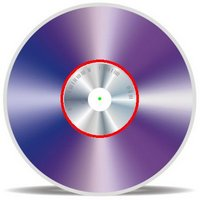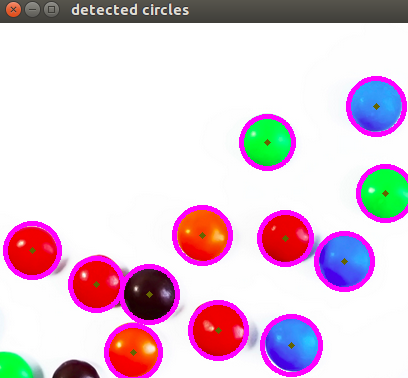OpenCV  3.4.2 Open Source Computer Vision
Hough Circle Transform

Prev Tutorial: Hough Line Transform

Next Tutorial: Remapping

## Goal

In this tutorial you will learn how to:

• Use the OpenCV function HoughCircles() to detect circles in an image.

## Theory

### Hough Circle Transform

• The Hough Circle Transform works in a roughly analogous way to the Hough Line Transform explained in the previous tutorial.
• In the line detection case, a line was defined by two parameters $$(r, \theta)$$. In the circle case, we need three parameters to define a circle:

$C : ( x_{center}, y_{center}, r )$

where $$(x_{center}, y_{center})$$ define the center position (green point) and $$r$$ is the radius, which allows us to completely define a circle, as it can be seen below:• For sake of efficiency, OpenCV implements a detection method slightly trickier than the standard Hough Transform: The Hough gradient method, which is made up of two main stages. The first stage involves edge detection and finding the possible circle centers and the second stage finds the best radius for each candidate center. For more details, please check the book Learning OpenCV or your favorite Computer Vision bibliography

#### What does this program do?

• Loads an image and blur it to reduce the noise
• Applies the Hough Circle Transform to the blurred image .
• Display the detected circle in a window.

## Explanation

The image we used can be found here

#### Proceed to apply Hough Circle Transform:

• with the arguments:
• gray: Input image (grayscale).
• circles: A vector that stores sets of 3 values: $$x_{c}, y_{c}, r$$ for each detected circle.
• HOUGH_GRADIENT: Define the detection method. Currently this is the only one available in OpenCV.
• dp = 1: The inverse ratio of resolution.
• min_dist = gray.rows/16: Minimum distance between detected centers.
• param_1 = 200: Upper threshold for the internal Canny edge detector.
• param_2 = 100*: Threshold for center detection.
• min_radius = 0: Minimum radius to be detected. If unknown, put zero as default.
• max_radius = 0: Maximum radius to be detected. If unknown, put zero as default.

#### Draw the detected circles:

You can see that we will draw the circle(s) on red and the center(s) with a small green dot

## Result

The result of running the code above with a test image is shown below: